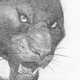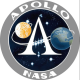# A new Approach Could Tease out the Connection Between Gravity and Quantum Mechanics

In physics, there are two main ways to model the universe. The first is the classical way. Classical models such as Newton’s laws of motion and Einstein’s theory of relativity assume that the properties of an object such as its position and motion are absolute. There are practical limits to how accurately we can measure an object’s path through space and time, but that’s on us. Nature knows their motion with infinite precision. Quantum models such as atomic physics assume that objects are governed by interactions. These interactions are probabilistic and indefinite. Even if we constrain an interaction to limited outcomes, we can never know the motion of an object with infinite precision, because nature doesn’t allow it.

These two theoretical worlds, the definite classical and indefinite quantum, each work extremely well. The classical for large, massive objects such as baseballs and planets, and the quantum for small, light objects such as atoms and molecules. But both of these approaches break down when we try to study massive but small things such as the interiors of black holes, or the observable universe in the earliest moments of the big bang. For that has all the properties of general relativity with all the properties of quantum theory. This theory is sometimes referred to as quantum gravity, but right now we don’t know it would work.

It’s difficult to study this theory because we don’t have any experiments to test it directly. But a new study proposes an experiment that could give us a glimpse of how quantum gravity might work.

The key is to have an object that is quantum in nature, but massive enough that classical gravity has an effect. To do this the team proposes using a super-cooled state of matter known as Bose-Einstein condensate. This occurs when certain groups of atoms are cooled so much that they effectively blur together in a single quantum state. If billions of atoms were cooled to a Bose-Einstein condensate, they would form a single quantum object with a mass roughly equal to that of a virus. Tiny, but massive enough for the effects of gravity to be studied.

The team proposes making such a condensate, then suspending it magnetically so that only gravity can interact with it. In their work, they show that if gravity works on a quantum level, then the shape of the condensate will shift slightly from its “weightless” Gaussian shape. If gravity only interacts on a classical level, then the condensate will remain Gaussian.

This approach could be done with our current technology. Unlike other proposed studies, this experiment would only rely on a basic property of quantum systems rather than more complex interactions such as entanglement. If the experiment can be performed, it could give us the first real look at the fundamental nature of quantum gravity.

Reference: Richard Howl, et al. “Non-Gaussianity as a Signature of a Quantum Theory of Gravity.” PRX Quantum 2.1 (2021): 010325.

## 2 Replies to “A new Approach Could Tease out the Connection Between Gravity and Quantum Mechanics”

1.Torbjörn Larsson says:

That is an interesting figure. It shows how it used to be a concern that general relativity could describe all sorts of topologies of curved space. It was, I believe, described as needing “a scaffold” to apply quantum field theory in so called semiclassical approximations.

But as it turned out cosmological space is flat, so the hatched arrow points back to classical quantum field theory. Maybe Wilczek was correct with his Feynman path integral “core theory” formulation of separating out the Lorentz metric as a factor – which happens when you quantize linearized gravity I believe – and gravity as an added quantum particle field.

Speculating further, “field lines” of mundane vector fields are mathematical tools without physical reality. So maybe these flat space constructions allow us to treat curvature of the gravity tensor field as mathematical convenience fictions as well.

2.Jim Krug says:

The introductory paragraphs to this article are fantastic- an excellent and understandable distinction between Newtonian and Quantum Physics.